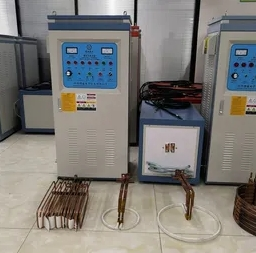### The induction coil is closely related to the frequency of the induction hardening equipment

The induction coil is closely related to the frequency of the induction hardening equipment

The induction coil is closely related to the inductance and frequency, and the factors affecting the induction coil mainly include the number of coils, the number of parallels, the length, the diameter, the diameter of the copper pipe, the spacing between the turns, the number of copper pipes, etc. Frequency quenching equipment should also pay attention to the inductance of the induction coil.

Generally speaking: the more turns, the greater the inductance, and the lower the frequency; otherwise, the higher; the longer the length, the greater the inductance, and the lower the frequency; otherwise, the higher;

The larger the diameter, the larger the inductance, the lower the frequency, and vice versa; the more parallels, the smaller the inductance, and the higher the frequency; otherwise, the lower;

The larger the turn spacing, the smaller the inductance, the higher the frequency, and vice versa; the larger the diameter of the copper tube, the smaller the inductance, the higher the frequency, and vice versa;

The more copper tubes, the smaller the inductance and the higher the frequency, and vice versa.